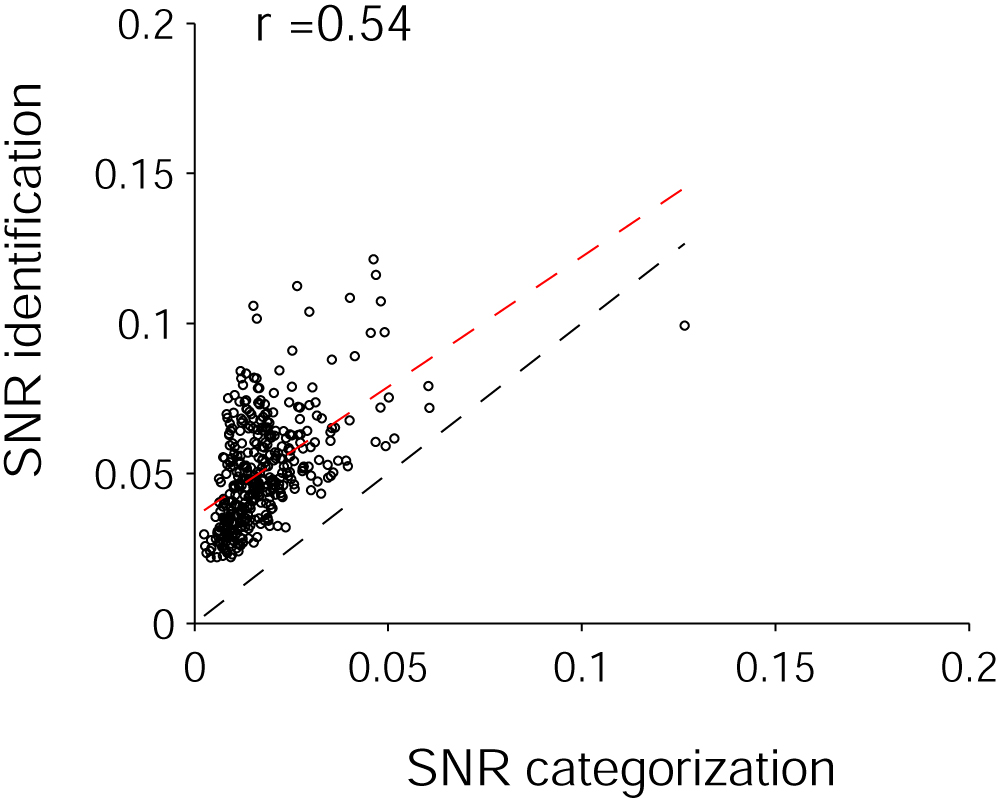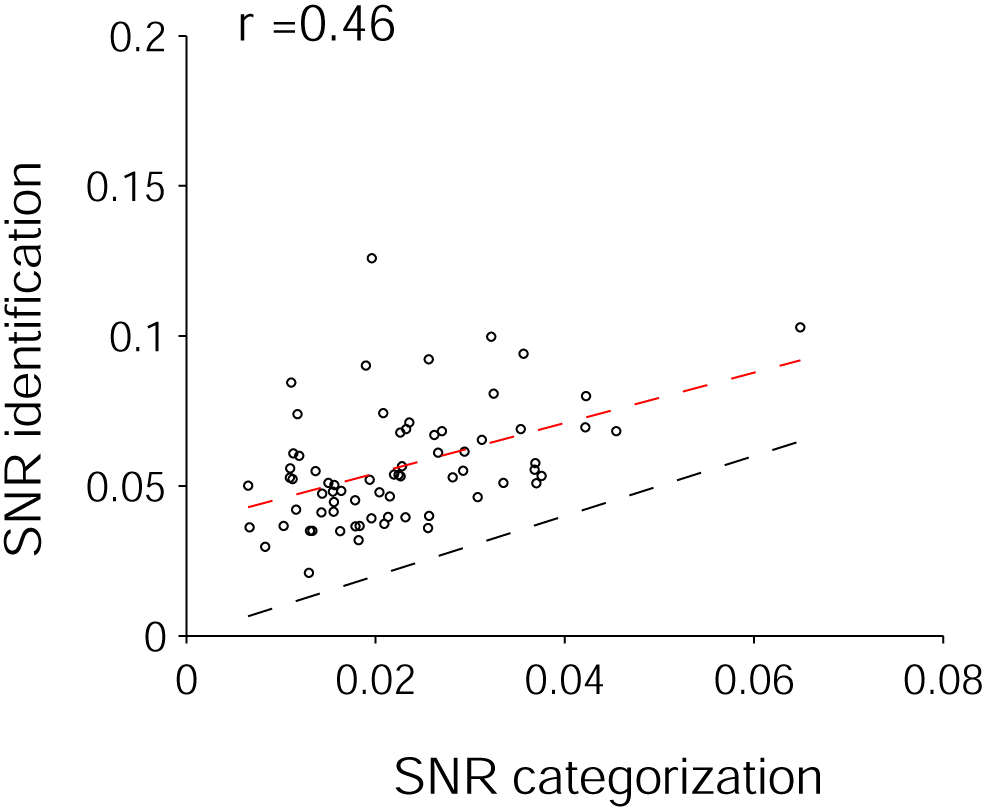The signal-to-noise ratio for categorization and identification are positively correlated

To further explore the neural code underlying putatively different types of information (identification vs categorization) expressed by the same population of neurons we used a standard variable selection technique (see Methods). Here we show the SNR for identification (y-axis) as a function of the SNR for categorization (x-axis) for each site (see below for definition of SNR). The black dashed line shows the diagonal y = x and the red dashed line represents a linear fit to the data (r = 0.54). The bottom follows the same format and corresponds to the data exploring invariance to object scale and position changes (r = 0.46).Parameters

Signal = MUA, Time interval = [100;300) ms, Bin size = 200 ms, Total number of sites = 367
The signal-to-noise ratio was defined as:where:
sSNRg is the signal-to-noise ratio for site s and group/picture g
<s_r_g> and s_s_g is the mean and standard deviation of the response of site s to group/picture g
<s_r_notg> and s_s_notg is the mean and standard deviation of the response of site s to stimuli not in group/picture g

Top